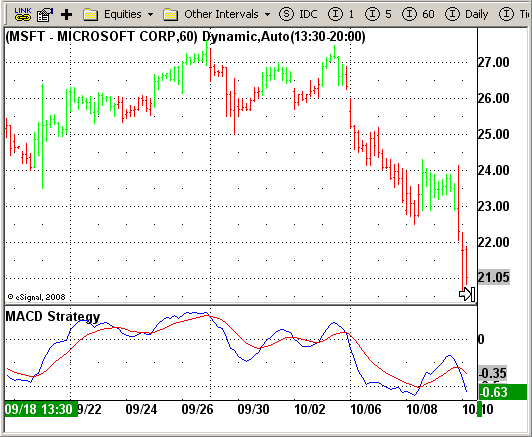# MACD Crossover

ICE Data Services -

MACDStrategy.efs
EFSLibrary - Discussion Board

File Name: MACDStrategy.efs

Description:
MACD Crossover

Formula Parameters:

Notes:

MACD V Moving Average Convergence Divergence. The MACD is calculated by subtracting a 26-day moving average of a security's price from a 12-day moving average of its price. The result is an indicator that oscillates above and below zero. When the MACD is above zero, it means the 12-day moving average is higher than the 26-day moving average.
This is bullish as it shows that current expectations (i.e., the 12-day moving average) are more bullish than previous expectations (i.e., the 26-day average). This implies a bullish, or upward, shift in the supply/demand lines. When the MACD falls below zero, it means that the 12-day moving average
is less than the 26-day moving average, implying a bearish shift in the supply/demand lines.
A 9-day moving average of the MACD (not of the security's price) is usually plotted on top of the MACD indicator. This line is referred to as the "signal" line. The signal line anticipates the convergence of the two moving averages (i.e., the movement of the MACD toward the zero line). Let's consider the rational behind this technique. The MACD is the difference between two moving averages of price. When the shorter-term moving average rises above the longer-term moving average (i.e., the MACD rises above zero), it means that investor expectations are becoming more bullish (i.e., there has been an upward shift in the supply/demand lines). By plotting a 9-day moving average of the MACD, we can see the changing of expectations (i.e., the shifting of the supply/demand lines) as they occur.

MACDStrategy.efsEFS Code:

```/*********************************
Provided By:
eSignal (Copyright c eSignal), a division of Interactive Data
Formula Script (EFS) is for educational purposes only and may be
modified and saved under a new file name.  eSignal is not responsible
for the functionality once modified.  eSignal reserves the right
to modify and overwrite this EFS file with each new release.

Description:
MACD Crossover

Version:            1.0  10/15/2008

Notes:
MACD – Moving Average Convergence Divergence. The MACD is calculated
by subtracting a 26-day moving average of a security's price from a
12-day moving average of its price. The result is an indicator that
oscillates above and below zero. When the MACD is above zero, it means
the 12-day moving average is higher than the 26-day moving average.
This is bullish as it shows that current expectations (i.e., the 12-day
moving average) are more bullish than previous expectations (i.e., the
26-day average). This implies a bullish, or upward, shift in the supply/demand
lines. When the MACD falls below zero, it means that the 12-day moving average
is less than the 26-day moving average, implying a bearish shift in the
supply/demand lines.
A 9-day moving average of the MACD (not of the security's price) is usually
plotted on top of the MACD indicator. This line is referred to as the "signal"
line. The signal line anticipates the convergence of the two moving averages
(i.e., the movement of the MACD toward the zero line).
Let's consider the rational behind this technique. The MACD is the difference
between two moving averages of price. When the shorter-term moving average rises
above the longer-term moving average (i.e., the MACD rises above zero), it means
that investor expectations are becoming more bullish (i.e., there has been an
upward shift in the supply/demand lines). By plotting a 9-day moving average of
the MACD, we can see the changing of expectations (i.e., the shifting of the
supply/demand lines) as they occur.

Formula Parameters:                     Default:

**********************************/

var bInit = false;

function preMain() {
setPriceStudy(false);
setColorPriceBars(true);
setDefaultPriceBarColor(Color.grey);
setStudyTitle("MACD Strategy");
setCursorLabelName("MACD", 0);
setCursorLabelName("SIGNAL", 1);
setDefaultBarFgColor(Color.blue, 0);
setDefaultBarFgColor(Color.red, 1);
}

var xMACD = null;
var xSignal = null;

function main() {

if (bInit == false) {
xMACD = macd(8, 16, 11);
xSignal = macdSignal(8, 16, 11);
bInit = true;
}

if (getCurrentBarCount() < 16) return;
if (getCurrentBarIndex() == 0) return;

if(xSignal.getValue(0) < xMACD.getValue(0) && !Strategy.isLong()) {
Strategy.doLong("Long", Strategy.CLOSE , Strategy.NEXTBAR);
}

if(xSignal.getValue(0) > xMACD.getValue(0) && !Strategy.isShort()) {
Strategy.doShort("Short", Strategy.CLOSE , Strategy.NEXTBAR);
}

if(Strategy.isLong())
setPriceBarColor(Color.lime);
else if(Strategy.isShort())
setPriceBarColor(Color.red);

return new Array(xMACD.getValue(0), xSignal.getValue(0));
}```